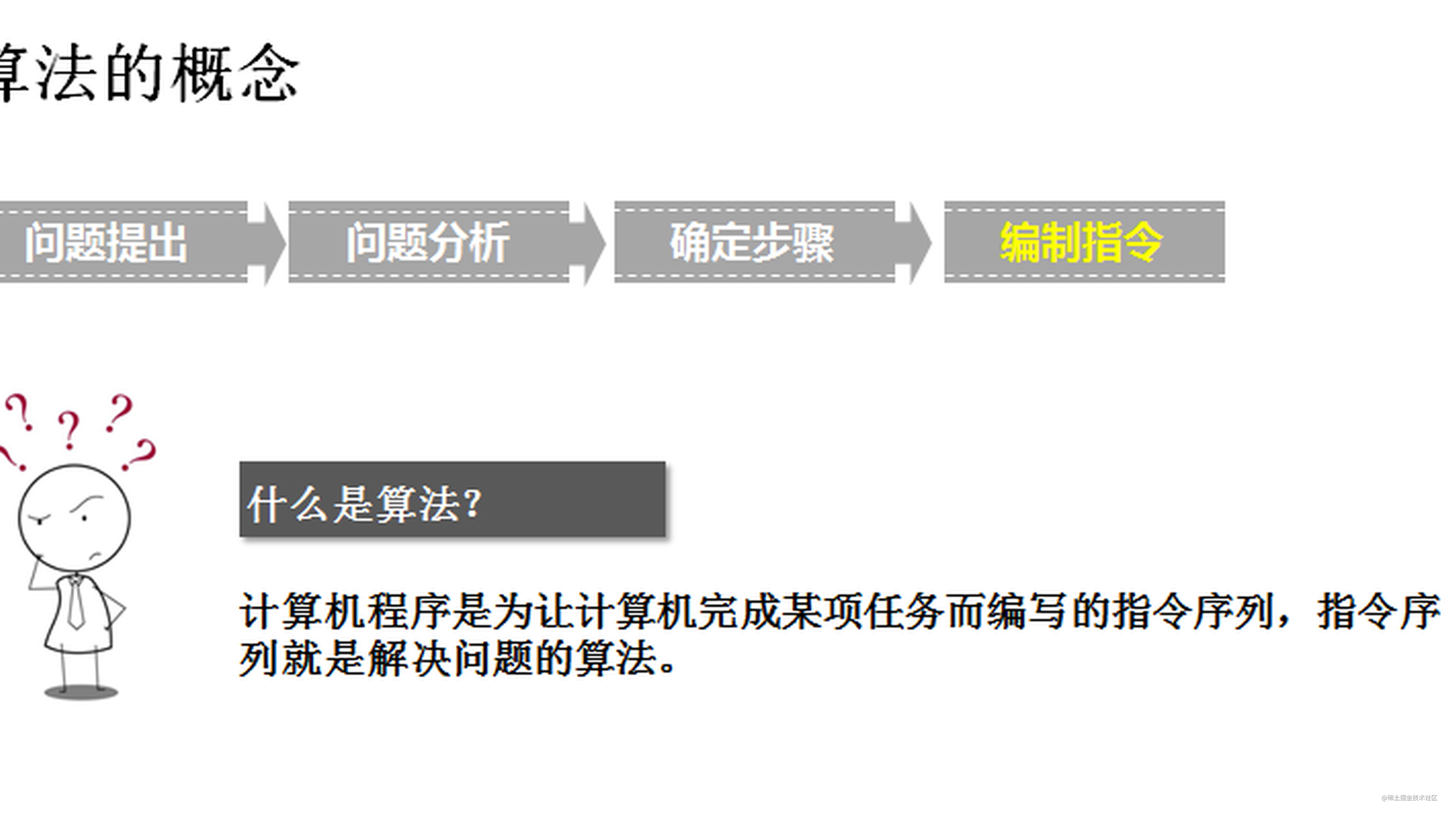# 【算法】6124. 第一个出现两次的字母（多语言实现）# 6124. 第一个出现两次的字母：

• 如果 `a`第二次 出现比 `b`第二次 出现在字符串中的位置更靠前，则认为字母 `a` 在字母 `b` 之前出现两次。
• `s` 包含至少一个出现两次的字母。

# 样例 1：

``````输入：
s = "abccbaacz"

"c"

字母 'a' 在下标 0 、5 和 6 处出现。
字母 'b' 在下标 1 和 4 处出现。
字母 'c' 在下标 2 、3 和 7 处出现。
字母 'z' 在下标 8 处出现。
字母 'c' 是第一个出现两次的字母，因为在所有字母中，'c' 第二次出现的下标是最小的。

# 样例 2：

``````输入：
s = "abcdd"

"d"

只有字母 'd' 出现两次，所以返回 'd' 。

# 提示：

• 2 <= s.length <= 100
• s 由小写英文字母组成
• s 包含至少一个重复字母

# 分析

• 面对这道算法题目，二当家的陷入了沉思。
• 按顺序遍历字符串，计数字母出现次数，第一次出现2次的就是结果。
• 关键是怎么计数，由于结果要的是出现2次，所以我们仅需要记录出现过的字母，用map，set，位运算，数组都可以。

# 题解

## rust

``````impl Solution {
pub fn repeated_character(s: String) -> char {
let mut ans = 'a';
let mut flag = vec![false; 26];

for &b in s.as_bytes().iter() {
if flag[(b - 97) as usize] {
ans = b as char;
break;
}
flag[(b - 97) as usize] = true;
}

ans
}
}

## go

``````func repeatedCharacter(s string) byte {
var ans byte
flag := make([]bool, 26)

for _, c := range s {
if flag[c-'a'] {
ans = byte(c)
break
}
flag[c-'a'] = true
}

return ans
}

## c++

``````class Solution {
public:
char repeatedCharacter(string s) {
char ans;
bool flag = {};
for (auto c: s) {
if (flag[c - 'a']) {
ans = c;
break;
}
flag[c - 'a'] = true;
}

return ans;
}
};

## java

``````class Solution {
public char repeatedCharacter(String s) {
char ans = 'a';
boolean[] flag = new boolean;

for (char c : s.toCharArray()) {
if (flag[c - 'a']) {
ans = c;
break;
}
flag[c - 'a'] = true;
}

return ans;
}
}

## python

``````class Solution:
def repeatedCharacter(self, s: str) -> str:
flag = set()
for c in s:
if c in flag:
return c